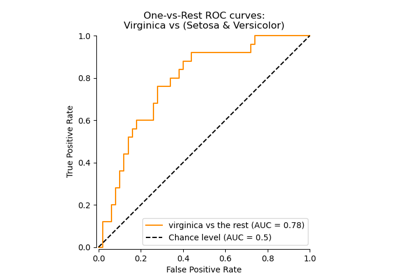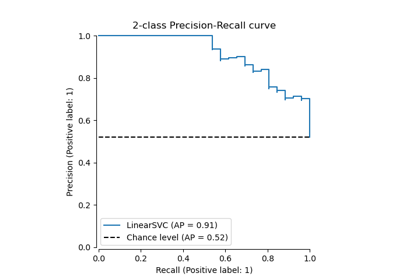# sklearn.preprocessing.label_binarize¶

sklearn.preprocessing.label_binarize(y, *, classes, neg_label=0, pos_label=1, sparse_output=False)[source]

Binarize labels in a one-vs-all fashion

Several regression and binary classification algorithms are available in scikit-learn. A simple way to extend these algorithms to the multi-class classification case is to use the so-called one-vs-all scheme.

This function makes it possible to compute this transformation for a fixed set of class labels known ahead of time.

Parameters
yarray-like

Sequence of integer labels or multilabel data to encode.

classesarray-like of shape [n_classes]

Uniquely holds the label for each class.

neg_labelint (default: 0)

Value with which negative labels must be encoded.

pos_labelint (default: 1)

Value with which positive labels must be encoded.

sparse_outputboolean (default: False),

Set to true if output binary array is desired in CSR sparse format

Returns
Ynumpy array or CSR matrix of shape [n_samples, n_classes]

Shape will be [n_samples, 1] for binary problems.

LabelBinarizer

class used to wrap the functionality of label_binarize and allow for fitting to classes independently of the transform operation

Examples

>>> from sklearn.preprocessing import label_binarize
>>> label_binarize([1, 6], classes=[1, 2, 4, 6])
array([[1, 0, 0, 0],
[0, 0, 0, 1]])


The class ordering is preserved:

>>> label_binarize([1, 6], classes=[1, 6, 4, 2])
array([[1, 0, 0, 0],
[0, 1, 0, 0]])


Binary targets transform to a column vector

>>> label_binarize(['yes', 'no', 'no', 'yes'], classes=['no', 'yes'])
array([,
,
,
])


## Examples using sklearn.preprocessing.label_binarize¶